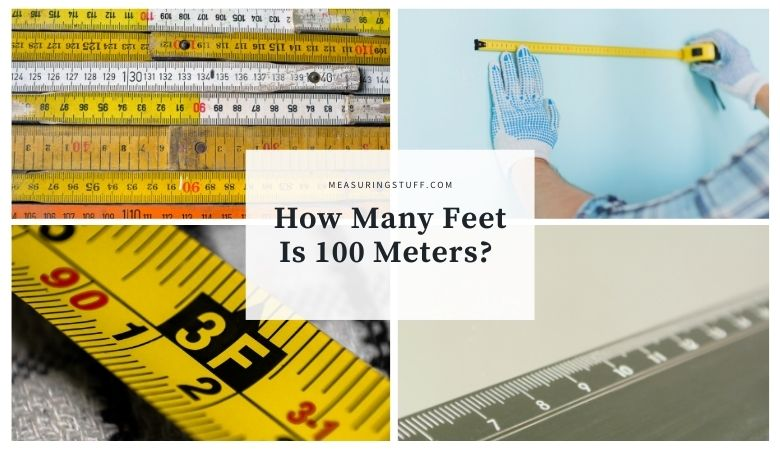# How Many Feet Is 100 Meters?

Last updated on September 4th, 2022 at 09:39 pm

100 meters equals 328.084 feet.

To convert meters into feet, you need to multiply by the conversion factor of 3.28084.

For example, if you want to know how many feet are in 100 meters, multiply the number of meters by 3.28084 to get the answer in feet.

100 meters x 3.28084 (conversion factor) = 328.084 feet.

The answer, which is 328.084, tells you that there are that many feet in 100 meters.

If you want to reverse the question and figure out how many meters can be made from a certain number of feet, you would divide the number of meters by 3.28084.

For example, if you have 200 feet, you can divide it by 3.28084 to get 60.960.

This means that there are 60.960 meters in 200 feet.

If you don’t feel like doing the math, use our online conversion calculator to convert different units of measurements and quickly give you the answer.

## Units of measurement conversion calculator

You can also use the following table to convert meters into feet.

## Feet measurement

A foot is a unit of length measurement that is equal to 12 inches or 0.305 meters.

CHECK OUT  How Many Grams Are In 5 Kilograms?

## Meters measurement

A Meter is part of the metric system of measurement which was created in France to have a way to measure length, mass, and volume. 1 meter is equal to 3 feet 3 inches in length.